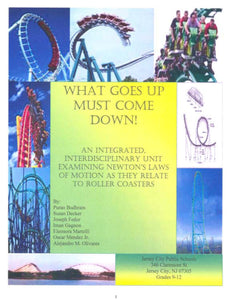# What goes up must come down!

• \$5.50

An integrated, interdisciplinary unit examining Newton's Laws of Motion as they relate to roller coasters. Space and Time Gravity is the most important force over large distances, and is responsible for large-scale motions in space. Gravity is the weakest of all the forces, but because it acts between all matters and because it decays at a moderate rate with distance, it is the most dominant force over large distances. Forces and Motion The most important equation in all of science is likely F=ma, which says that when you push on an object, it accelerates. Push on a massive object, and it accelerates slowly. Push harder, and it accelerates faster. The most prevalent force on our planet's surface is the gravitational pull of Earth's mass. As a result, the most prevalent kind of motion is downward acceleration (i.e., things fall downhill). Matter and Energy As Einstein showed, gravity is better viewed as a distortion of the fabric of space by matter. Very massive objects, like black holes and galaxies create very large "warping" of the spatial dimensions of space. This explains why light from distant galaxies is bent around intervening galaxies. Forces and Motion Kinetic energy of motion: The most common type of KE is the motion of macroscopic objects. Kinetic energy is calculated as one half of the product of the mass of the object times the square of the object's velocity. Newton was one of the most influential scientists of the seventeenth century. He discovered three basic laws that explain all aspects of motion. To build a roller coaster it is very useful to know something about these famous laws. These are some of the essential concepts in this unit to discuss with the students, to understand the functioning of the roller coasters: Acceleration Potential Energy Energy Kinetic energy Force Gravitational Force Momentum Sir Isaac Newton Velocity Work